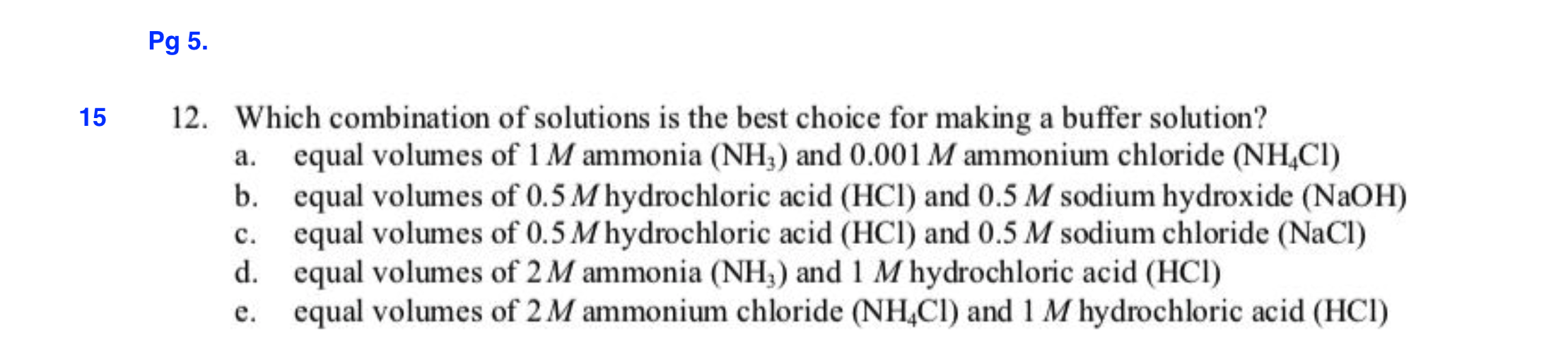# Pg 5.12. Which combination of solutions is the best choice for making a buffer solution?15equal volumes of 1 M ammonia (NH) and 0.001 M ammonium chloride (NHCI)equal volumes of 0.5 M hydrochloric acid (HCl) and 0.5 M sodium hydroxide (NaOH)equal volumes of 0.5 M hydrochloric acid (HCl) and 0.5 M sodium chloride (NaCl)equal volumes of 2M ammonia (NH) and 1 M hydrochloric acid (HCI)equal volumes of 2 M ammonium chloride (NH,CI) and 1 M hydrochloric acid (HCI)а.b.с.d.е.

Question
77 views

Which combination of solutions is the best for making a buffer solution?

*Please show the reactions that are taking place. That's mainly what I'm having trouble with. Please also explain what happens to the spectator ions.help_outlineImage TranscriptionclosePg 5. 12. Which combination of solutions is the best choice for making a buffer solution? 15 equal volumes of 1 M ammonia (NH) and 0.001 M ammonium chloride (NHCI) equal volumes of 0.5 M hydrochloric acid (HCl) and 0.5 M sodium hydroxide (NaOH) equal volumes of 0.5 M hydrochloric acid (HCl) and 0.5 M sodium chloride (NaCl) equal volumes of 2M ammonia (NH) and 1 M hydrochloric acid (HCI) equal volumes of 2 M ammonium chloride (NH,CI) and 1 M hydrochloric acid (HCI) а. b. с. d. е. fullscreen
check_circle

Step 1

A buffer solution contains a mixture of a weak base/acid and it’s conjugate acid/base. In a good buffer solution, the concentration of the weak base and the conjugate acid are equal. The correct answer is option d.

Step 2

On the mixing of equal volumes of 2 M NH3 and 1 M HCl, only half of the NH3 reacts with all the HCl to produce NH4Cl. The reaction of NH3 and HCl is as follows:

Step 3

On the addition of equal volumes of acid and base, the concentration of NH3 and HCl in the mixture are 1 M and 0.5 M, respectively. On the reaction of NH3 with HCl, half of the ammonia gets converted into ammonium chloride. After the reaction, the final solution contains 0.5 M NH3 and 0....

### Want to see the full answer?

See Solution

#### Want to see this answer and more?

Solutions are written by subject experts who are available 24/7. Questions are typically answered within 1 hour.*

See Solution
*Response times may vary by subject and question.
Tagged in